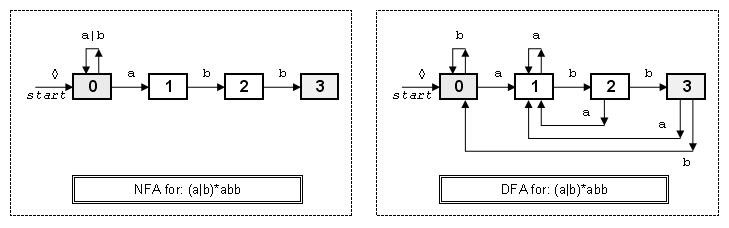# Compiler Design 

2009 Oct 18

 Compiler Phases Lexical Analysis Syntactic Analysis Intermediate Code Generation Code Optimization Code Generation Support Table Management Error Handling Program Data Data - declarations Heap - dynamic Stack - code linkages Functions Blocks Declarations Statments Control Flow Expressions Data references Function calls

### Lexical Finite State Machines

A FSM can be either deterministic (DFA) or nondeterministic (NFA). For the regular expression: (a|b)*abb it is easy to construct the following NFA (where the green state is the single start state and blue states are final or accepting states (in general there can be more than one). Transitions are labeled with the character set that they recognize. A special symbol, , is used to label an empty transition; i.e. one which takes place without an input character being consumed. It is always possible to construct a DFA for any NFA, although the number of states in the resulting DFA could be exponential.The algorithm to convert a NFA to a DFA is often called Subset Construction.

Input: An NFA, N, specified as a list of states (with special start state 0), where each state has a list of input characters (including ). Associated with this list of input characters is the list of states, for each input character, that input will (nondeterninistically) transition to. For the above example this is:

```0; a{0, 1}, b{0}
1; b{2}
2; b{3}
3+; // the plus marks state 3 as an accepting state
```
Output: A DFA, D, also accepting N's language.

Algorithm: Each state of D is a set of states which N could be in after reading some sequence of input symbols, thus simulating in parallel all possible moves, or state transitions, N can make on a given input string. The initial state of D is the set of consisting of s0 (from N along with all states of N reachable by transitions.

The function closure(s) is defined to be the set of states of N build by appling the following rules:

1. s is added to closure(s)
2. if t is in closure(s), and there is a transition labeled from t to u then u is added to closure(s) if u is not already there. Rule 2 is repeated until no more states can be added to closure(s).

Thus closure(s) is just the set of states that can reached from s on transitions alone.

The states of D and their transitions are constructed as follows. Initially, let closure(s0) be a state of D - this is the start state of D. Initially every state of D is unmarked. Then perform the subset algorithm:

```subset() {
while there is an unmarked state x={s1, s2, ... } of D do {
mark x;
for each input symbol a {
Let T be the set of states to which there is a transition on a
from state si in x;
y := closure(T);
if y has not yet been added to set of states of D
then make y an unmarked state of D;
Add a transition from x to y labeled a if not
```Left  Associative: ( ( a + b ) + c ) )